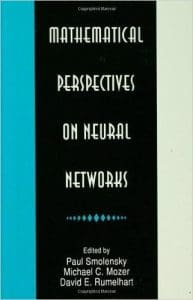# Mathematical Perspectives on Neural NetworksRecent years have seen an explosion of new mathematical results on learning and processing in neural networks. This body of results rests on a breadth of mathematical background which even few specialists possess. In a format intermediate between a textbook and a collection of research articles, this book has been assembled to present a sample of these results, and to fill in the necessary background, in such areas as computability theory, computational complexity theory, the theory of analog computation, stochastic processes, dynamical systems, control theory, time-series analysis, Bayesian analysis, regularization theory, information theory, computational learning theory, and mathematical statistics.

Mathematical models of neural networks display an amazing richness and diversity. Neural networks can be formally modeled as computational systems, as physical or dynamical systems, and as statistical analyzers. Within each of these three broad perspectives, there are a number of particular approaches. For each of 16 particular mathematical perspectives on neural networks, the contributing authors provide introductions to the background mathematics, and address questions such as:

• Exactly what mathematical systems are used to model neural networks from the given perspective?